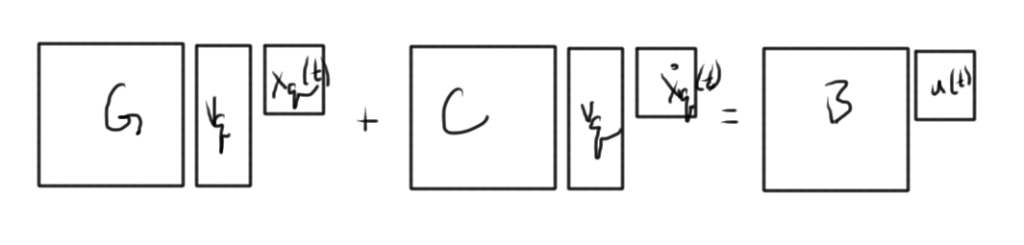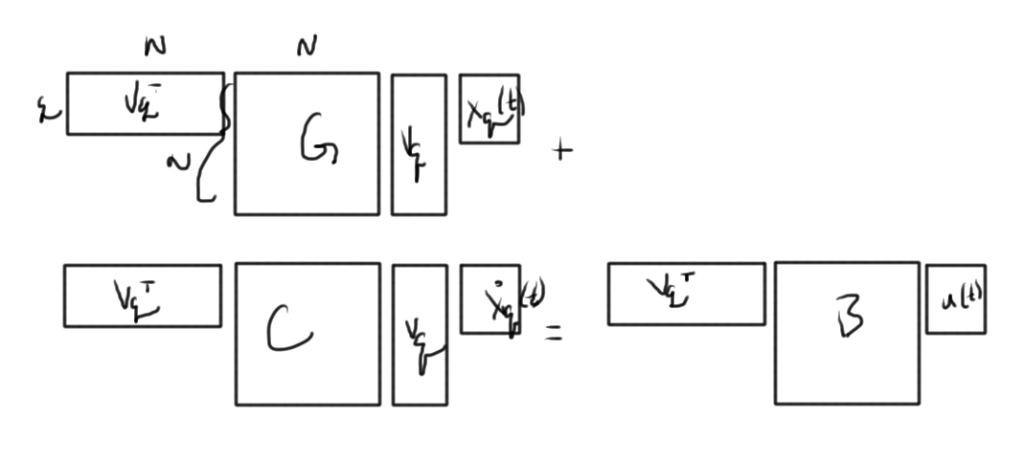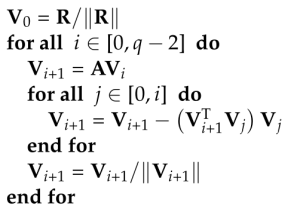## Disclaimer

Peeter’s lecture notes from class. These may be incoherent and rough.

## Model order reduction (cont).

An approximation of the following system is sought

\begin{equation}\label{eqn:multiphysicsL19:20}
\BG \Bx(t) + C \dot{\Bx}(t) = B \Bu(t)
\end{equation}
\begin{equation}\label{eqn:multiphysicsL19:40}
\By(t) = \BL^\T \Bx(t).
\end{equation}

The strategy is to attempt to find a $$N \times q$$ projector $$\BV$$ of the form

\begin{equation}\label{eqn:multiphysicsL19:60}
\BV =
\begin{bmatrix}
\Bv_1 & \Bv_2 & \cdots & \Bv_q
\end{bmatrix}
\end{equation}

so that the solution of the constrained q-variable state vector $$\Bx_q$$ is sought after letting

\begin{equation}\label{eqn:multiphysicsL19:80}
\Bx(t) = \BV \Bx_q(t).
\end{equation}

## Moment matching

\begin{equation}\label{eqn:multiphysicsL19:100}
\begin{aligned}
\BF(s)
&= \lr{ \BG + s \BC }^{-1} \BB \\
&= \BM_0 + \BM_1 s + \BM_2 s^2 + \cdots + \BM_{q-1} s^{q-1} + M_q s^q + \cdots
\end{aligned}
\end{equation}

The reduced model is created such that

\begin{equation}\label{eqn:multiphysicsProblemSet3b:120}
\BF_q(s)
=
\BM_0 + \BM_1 s + \BM_2 s^2 + \cdots + \BM_{q-1} s^{q-1} + \tilde{\BM}_q s^q.
\end{equation}

Form an $$N \times q$$ projection matrix

\begin{equation}\label{eqn:multiphysicsL19:140}
\BV_q \equiv
\begin{bmatrix}
\BM_0 & \BM_1 & \cdots & \BM_{q-1}
\end{bmatrix}
\end{equation}

With the substitution of fig. 1, becomesThis is a system of $$N$$ equations, in $$q$$ unknowns. A set of moments from the frequency domain have been used to project the time domain system. This relies on the following unproved theorem (references to come)

### Theorem

If $$\text{span}\{ \Bv_q \} = \text{span} \{ \BM_0, \BM_1, \cdots, \BM_{q-1} \}$$, then the reduced model will match the first $$q$$ moments.

Left multiplication by $$\BV_q^\T$$ yields fig. 2.This is now a system of $$q$$ equations in $$q$$ unknowns.

With

\begin{equation}\label{eqn:multiphysicsL19:160}
\BG_q = \BV_q^\T \BG \BV_q
\end{equation}
\begin{equation}\label{eqn:multiphysicsL19:180}
\BC_q = \BV_q^\T \BC \BV_q
\end{equation}
\begin{equation}\label{eqn:multiphysicsL19:200}
\BB_q = \BV_q^\T \BB
\end{equation}
\begin{equation}\label{eqn:multiphysicsL19:220}
\BL_q^\T = \BL^\T \BV_q
\end{equation}

the system is reduced to

\begin{equation}\label{eqn:multiphysicsL19:240}
\BG_q \Bx_q(t) + \BC_q \dot{\Bx}_q(t) = \BB_q \Bu(t).
\end{equation}
\begin{equation}\label{eqn:multiphysicsL19:260}
\By(t) \approx \BL_q^\T \Bx_q(t)
\end{equation}

### Moments calculation

Using
\begin{equation}\label{eqn:multiphysicsL19:300}
\lr{ \BG + s \BC }^{-1} \BB = \BM_0 + \BM_1 s + \BM_2 s^2 + \cdots
\end{equation}

thus
\begin{equation}\label{eqn:multiphysicsL19:320}
\begin{aligned}
\BB &=
\lr{ \BG + s \BC }
\BM_0 +
\lr{ \BG + s \BC }
\BM_1 s +
\lr{ \BG + s \BC }
\BM_2 s^2 + \cdots \\
&=
\BG \BM_0
+ s \lr{ C \BM_0 + \BG \BM_1 }
+ s^2 \lr{ C \BM_1 + \BG \BM_2 }
+ \cdots
\end{aligned}
\end{equation}

Since $$\BB$$ is a zeroth order matrix, setting all the coefficients of $$s$$ equal to zero provides a method to solve for the moments

\begin{equation}\label{eqn:multiphysicsL19:340}
\begin{aligned}
\BB &= \BG \BM_0 \\
-\BC \BM_0 &= \BG \BM_1 \\
-\BC \BM_1 &= \BG \BM_2 \\
\end{aligned}
\end{equation}

The moment $$\BM_0$$ can be found with LU of $$\BG$$, plus the forward and backward substitutions. Proceeding recursively, using the already computed LU factorization, each subsequent moment calculation requires only one pair of forward and backward substitutions.

Numerically, each moment has the exact value

\begin{equation}\label{eqn:multiphysicsL19:360}
\BM_q = \lr{- \BG^{-1} \BC }^q \BM_0.
\end{equation}

As $$q \rightarrow \infty$$, this goes to some limit, say $$\Bw$$. The value $$\Bw$$ is related to the largest eigenvalue of $$-\BG^{-1} \BC$$. Incidentally, this can be used to find the largest eigenvalue of $$-\BG^{-1} \BC$$.

The largest eigenvalue of this matrix will dominate these factors, and can cause some numerical trouble. For this reason it is desirable to avoid such explicit moment determination, instead using implicit methods.

The key is to utilize the theorem above, and look instead for an alternate basis $$\{ \Bv_q \}$$ that also spans $$\{ \BM_0, \BM_1, \cdots, \BM_q \}$$.

### Space generate by the moments

Write

\begin{equation}\label{eqn:multiphysicsL19:380}
\BM_q = \BA^q \BR,
\end{equation}

where in this case

\begin{equation}\label{eqn:multiphysicsL19:400}
\begin{aligned}
\BA &= – \BG^{-1} \BC \\
\BR &= \BM_0 = -\BG \BB
\end{aligned}
\end{equation}

The span of interest is

\begin{equation}\label{eqn:multiphysicsL19:420}
\text{span} \{ \BR, \BA \BR, \BA^2 \BR, \cdots, \BA^{q-1} \BR \}.
\end{equation}

Such a sequence is called a Krylov subspace. One method to compute such a basis, the Arnoldi process, relies on Gram-Schmidt orthonormalization methods:Some numerical examples and plots on the class slides.